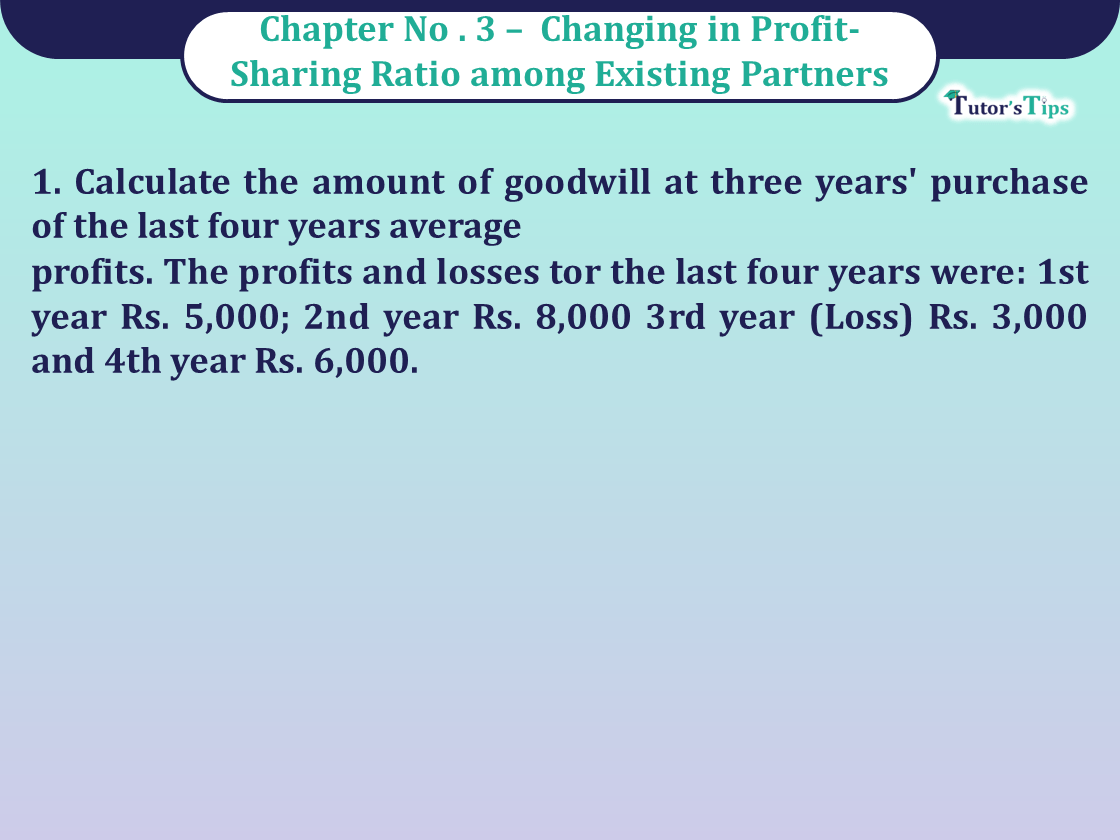# Question 1 Chapter 3 of Class 12 Part – 1 VK PublicationQuestion 1 Chapter 3 of Class 12 Part - 1 VK Publication

Question 1 Chapter 3 of Class 12 Part – 1

1. Calculate the amount of goodwill at three years’ purchase of the last four years average
profits. The profits and losses tor the last four years were: 1st year Rs. 5,000; 2nd year Rs. 8,000 3rd year (Loss) Rs. 3,000 and 4th year Rs. 6,000.

## The solution of Question 1 Chapter 3 of Class 12 Part – 1: –

 Average Profit = Total Profits Number of years = 5,000+8,000-3,000+6,000 4 = 16,000 4 = 4,000

Goodwill = Average Profit x Number of Years’ Purchase
= 4,000 x 3 = Rs. 12,000.

Comment if you have any questions.

Also, Check out the solved question of previous Chapters: –